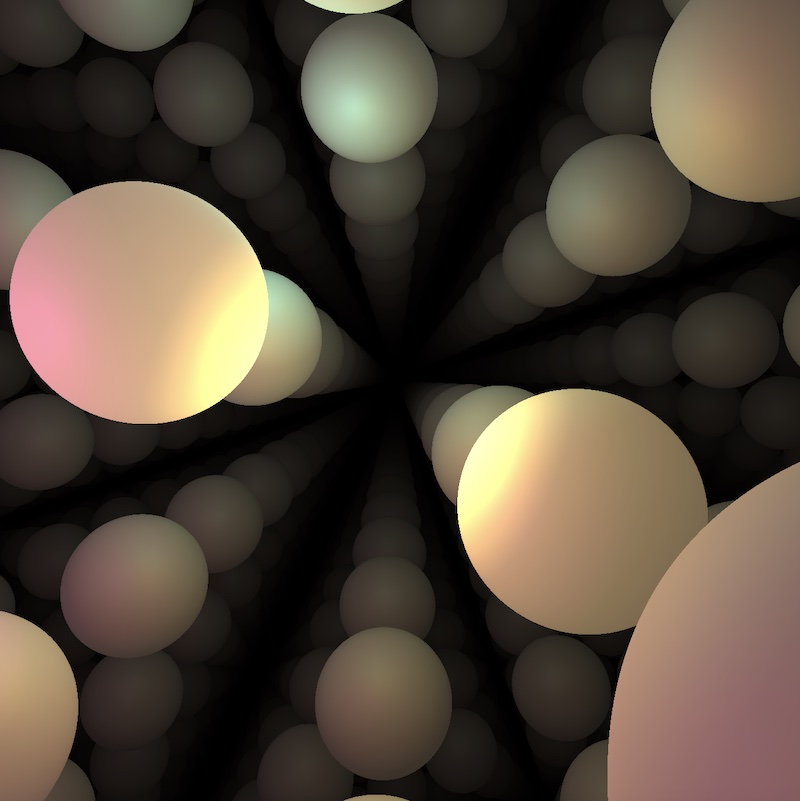# The euclidean space $\mathbb E^3$

## What is $\mathbb E^3$?

The euclidean space corresponds to the “usual” 3D geometry we learn at school. It is the geometry of the flat real 3-dimensional vector space $\mathbb R ^3$.

Click on the button below to reveal a concrete model of $\mathbb E^3$.

As a set of points, $\mathbb E^3$ is the usual 3-dimensional space $X = \mathbb R^3$ with coordinates $(x,y,z)$. Its riemanian metric is $$ds^2 = dx^2 + dy^2 + dz^2 + dw^2.$$ Its isometry group is the affine group $\mathbb R^3 \rtimes O(3)$, where $\mathbb R^3$ corresponds to the translation group and $O(3)$ to the stabilizer of a point.

## Some views of $\mathbb{E}^3$

Warning: Some of the real-time simulations below requires a powerful graphic card. If your computer is not fast enough, you can reduce the size of your browser window. Click on the button below to reveal the fly commands.

The default controls to fly in the scene are the following. You can choose your keyboard in the Option controls pannel in the top right corner of the window

Command QWERTY keyboard AZERTY keyboard
Yaw left a q
Yaw right d d
Pitch up w z
Pitch down s s
Roll left q a
Roll right e e
Move forward arrow up arrow up
Move backward arrow down arrow down
Move to the left arrow left arrow left
Move the the right arrow right arrow right
Move upwards ' ù
Move downwards / =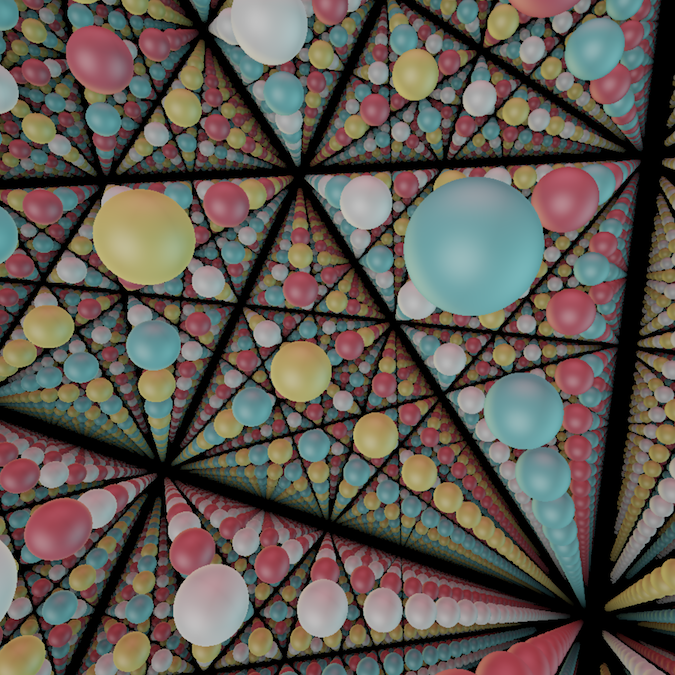##### Spheres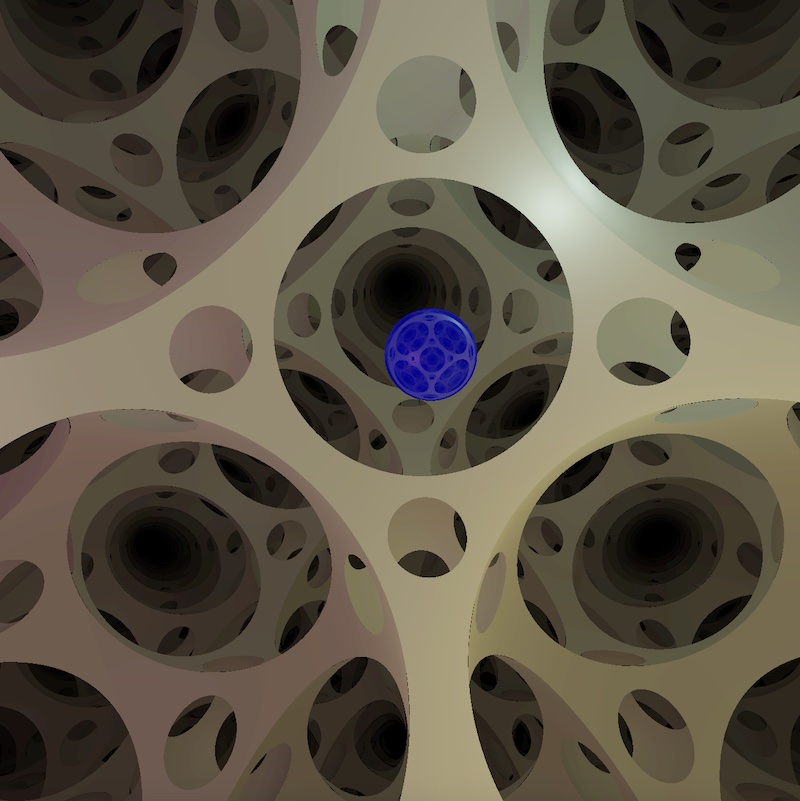##### The Cubical 3-Torus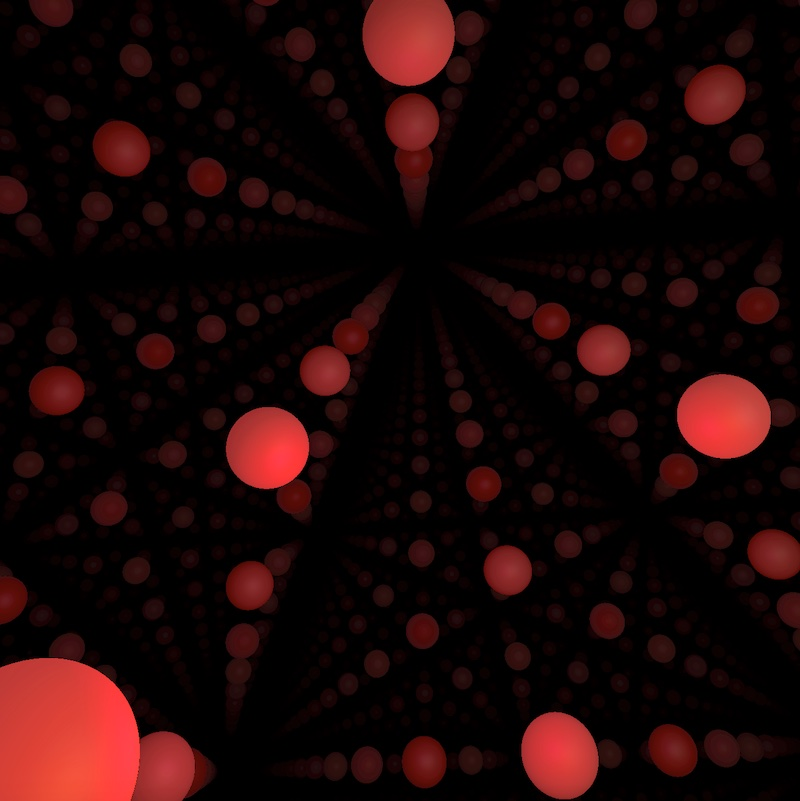##### A 3-Torus undergoing a Dehn Twist

(Backup you start inside a ball)

HD pictures of $\mathbb{E}^3$ can be found in the gallery

## Features of $\mathbb{E}^3$

Euclidean space is very familiar to us from everyday life, and so we have little to learn of its features from computer simulation. Nonetheless, a select few simulations below are included to allow comparison with other geometries.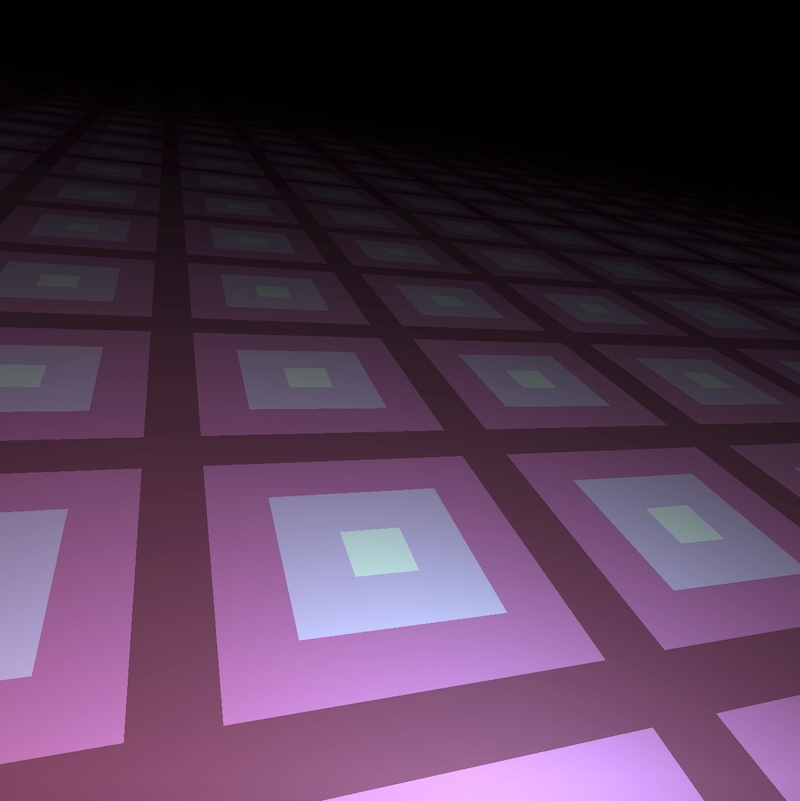##### Infinite Planes Take up Half your View##### Asymptotically Half a Sphere is Visible